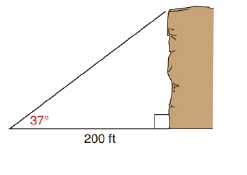Chapter 11.2, Problem 32EElementary Geometry For College St...

7th Edition
Alexander + 2 others
ISBN: 9781337614085

Solutions

Chapter
SectionElementary Geometry For College St...

7th Edition
Alexander + 2 others
ISBN: 9781337614085
Textbook Problem

In Exercise 29 to 37, angle measures should be given to the nearest degree; distances should be given to the nearest tenth of a unit.At a point 200 ft. from the base of a cliff, Journey sees the top of the cliff through an angle of elevation of 37 ∘ . How tall is the cliff?To determine

To find:

To find the height of the cliff by using the following condition,

“At a point 200 ft. from the base of a cliff, Journey sees the top of the cliff through an angle of elevation of 37.”

Explanation

Consider the following figure,

General formula for cosine ratio and sin ratio is given below,

From the given figure the value x ft. is the hypotenuse value since it is opposite to right angle.

Then y ft. is the length of the leg opposite to 37 and 200 ft. is the length of the leg adjacent to 37.

Therefore,

cos37=200xx=200cos37

Using a graphing calculator in degree mode, follow the following key sequence,

cos(37)enter0.7986

Substitute the value of cos37 in the above equation to get the following,

Still sussing out bartleby?

Check out a sample textbook solution.

See a sample solution

The Solution to Your Study Problems

Bartleby provides explanations to thousands of textbook problems written by our experts, many with advanced degrees!

Get Started

A sample of n = 9 scores has X = 108. What is the sample mean?

Essentials of Statistics for The Behavioral Sciences (MindTap Course List)

Expand each expression in Exercises 122. (x2+x1)(2x+4)

Finite Mathematics and Applied Calculus (MindTap Course List)

Sometimes, Always, or Never: If an ≥ bn ≥ 0 for all n and {bn} diverges, then {an} diverges.

Study Guide for Stewart's Single Variable Calculus: Early Transcendentals, 8th

For , which vector, a, b, c, or d, best represents F(1, 1)? a b c d

Study Guide for Stewart's Multivariable Calculus, 8th